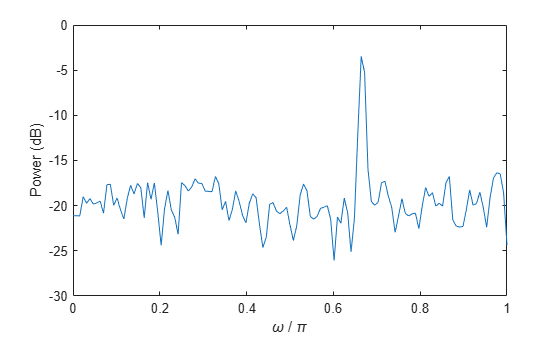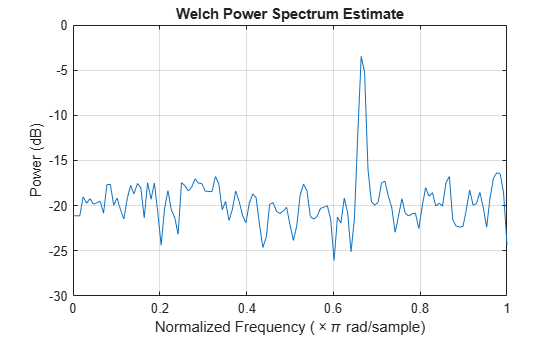# pow2db

Convert power to decibels

## Syntax

ydb = pow2db(y)

## Description

example

ydb = pow2db(y) expresses in decibels (dB) the power measurements specified in y. The relationship between power and decibels is ydb = 10 log10(y).

## Examples

collapse all

Generate 1024 samples of a noisy sinusoid having a normalized frequency of $2\pi /3$ rad/sample. Estimate the power spectrum of the signal using pwelch. Express the estimate in decibels and plot it.

n = 0:1024-1; x = cos(2*pi*n/3) + randn(size(n)); [pxx,w] = pwelch(x,'power'); dB = pow2db(pxx); plot(w/pi,dB) xlabel('\omega / \pi') ylabel('Power (dB)')Repeat the computation using pwelch without output arguments.

pwelch(x,'power')## Input Arguments

collapse all

Input array, specified as a scalar, vector, matrix, or N-D array. When y is nonscalar, pow2db is an element-wise operation.

Data Types: single | double

## Output Arguments

collapse all

Power measurements in decibels, returned as a scalar, vector, matrix, or N-D array of the same size as y.

## Version History

Introduced in R2007b